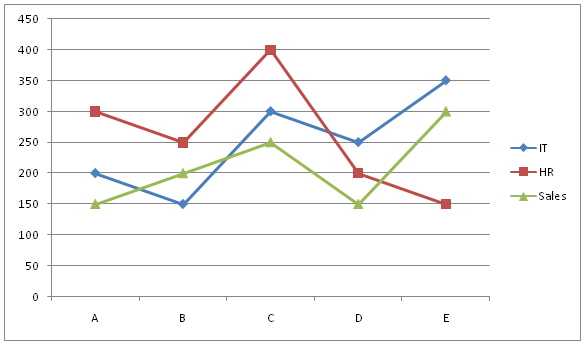# SBI Clerk Prelims 2021 Quantitative Aptitude Questions (Day-52)

Dear Aspirants, Our IBPS Guide team is providing new series of Quantitative Aptitude Questions for SBI Clerk Prelims 2020 so the aspirants can practice it on a daily basis. These questions are framed by our skilled experts after understanding your needs thoroughly. Aspirants can practice these new series questions daily to familiarize with the exact exam pattern and make your preparation effective.

Start Quiz

Missing number series

Directions (01-05): What value should come in the place of (?) in the following number series?

1) 15, 120, ?, 5040, 25200, 100800

A.840

B.240

C.360

D.480

E.720

2) 125, 148, 174, 203, 235, ?

A.260

B.250

C.270

D.280

E.290

3) 21, 55, 100, ?, 231, 321

A.128

B.138

C.148

D.158

E.178

4) 24, 73, 109, 134, 150, ?

A.160

B.154

C.159

D.170

E.164

5) 12, 18, 45, 67.5, ?, 253.125

A.145.85

B.152.50

C.168.75

D.172.25

E.180.125

Line graph

Directions (06-10): Study the following information carefully and answer the given questions.

The given line graph shows the number of employees in three different departments in five different companies.6) What is the difference between the total number of HR and sales department employees from all the companies together?

A.200

B.250

C.300

D.150

E.100

7) In IT department, which company has 40% more employees than company D?

A.A

B.B

C.C

D.E

E.None

8) Number of employees in HR department from E is approximately what percent of the total number of employees from C in all the departments together?

A.12%

B.16%

C.20%

D.8%

E.4%

9) What is the ratio of the total number of employees from D in all the departments together to the total number of employees from A in all the departments together?

A.11:12

B.12:13

C.13:14

D.14:15

E.15:16

10) What is the average number of employees in IT departments from all the companies together?

A.100

B.150

C.200

D.250

E.300

15 * 8 = 120

120 * 7 = 840

840 * 6 = 5040

5040 * 5 = 25200

25200 * 4 = 100800

125 + 23 = 148

148 + 26 = 174

174 + 29 = 203

203 + 32 = 235

235 + 35 = 270

21 + 42 + 18 = 55

55 + 52 + 20 = 100

100 + 62 + 22 = 158

158 + 72 + 24 = 231

231 + 82 + 26 = 321

24 + 72 =73

73 + 62 =109

109 + 52 =134

134 + 42 =150

150 + 32 =159

12 * 1.5 = 18

18 * 2.5 = 45

45 * 1.5 = 67.5

67.5 * 2.5 = 168.75

168.75 * 1.5 = 253.125

HR = 300 + 250 + 400 + 200 + 150 = 1300

Sales = 150 + 200 + 250 + 150 + 300 = 1050

Difference = 1300 – 1050 = 250

Total number of employees in IT department of D = 250

Total number of employees in IT department of A = 200/250 * 100 = 80%

Total number of employees in IT department of B = 150/250 * 100 = 60%

Total number of employees in IT department of C = 300/250 * 100 = 120%

Total number of employees in IT department of E = 350/250 * 100 = 140%

Company E has 40% more employees than company D.

Required percentage = [150/(300 + 400 + 250)] * 100 = 16%

Required ratio = (250 + 200 + 150):(200 + 300 + 150)

= 600:650

= 12:13